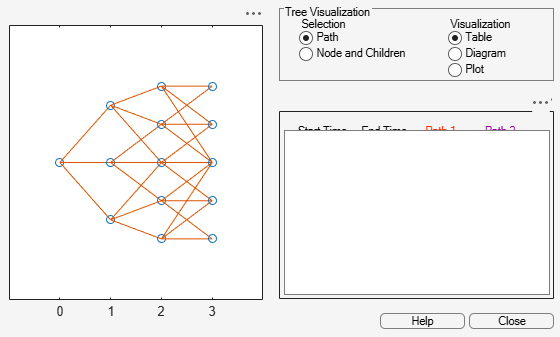# trintreepath

Entries from node of recombining trinomial tree

## Syntax

``Values = trintreepath(TrinTree,BranchList)``

## Description

example

````Values = trintreepath(TrinTree,BranchList)` extracts entries of a node of a recombining trinomial tree. The node path is described by the sequence of branchings taken, starting at the root. The top branch is number 1, the middle branch is 2, and the bottom branch is 3. Set the branch sequence to 0 to obtain the entries at the root node. ```

## Examples

collapse all

Create a `HW` tree by loading the example file.

```load deriv.mat; FwdRates = trintreepath(HWTree, [1 2 3])```
```FwdRates = 4×1 1.0279 1.0528 1.0652 1.0591 ```

This returns the rates at the tree nodes located by starting at 0, taking the up branch at the first node, the middle branch at the second node, and finally the bottom branch at the third node.

You can visualize this with the `treeviewer` function.

`treeviewer(HWTree)`## Input Arguments

collapse all

Recombining price or interest-rate trinomial tree, specified as a struct that is created using one of the following functions:

Data Types: `struct`

Number of paths (`NUMPATHS`) by path length (`PATHLENGTH`), specified as a matrix containing the sequence of branchings.

Data Types: `double`

## Output Arguments

collapse all

Retrieved entries of a recombining tree, returned a number of values (`NUMVALS`)-by-`NUMPATHS` matrix.

## Version History

Introduced before R2006a